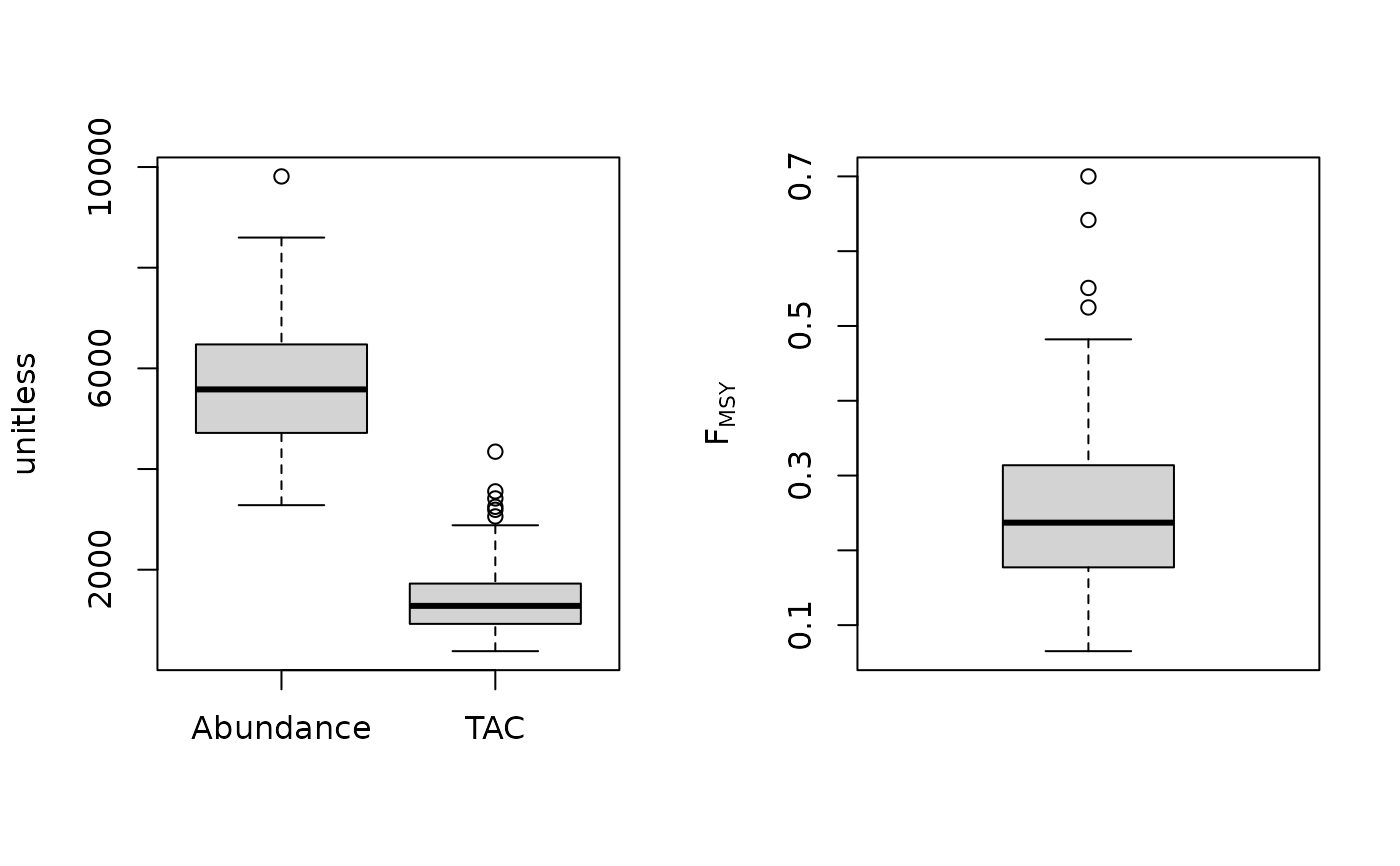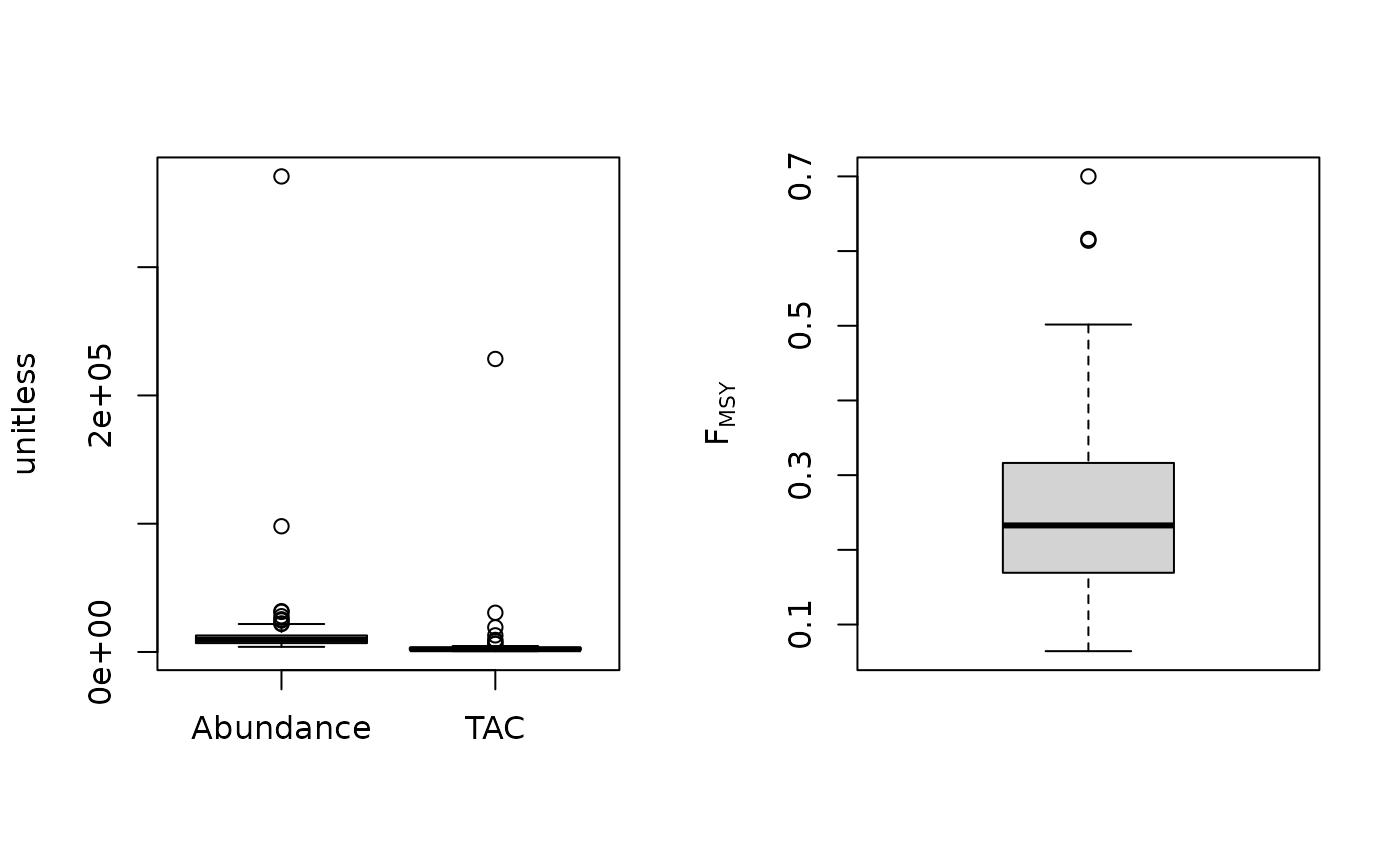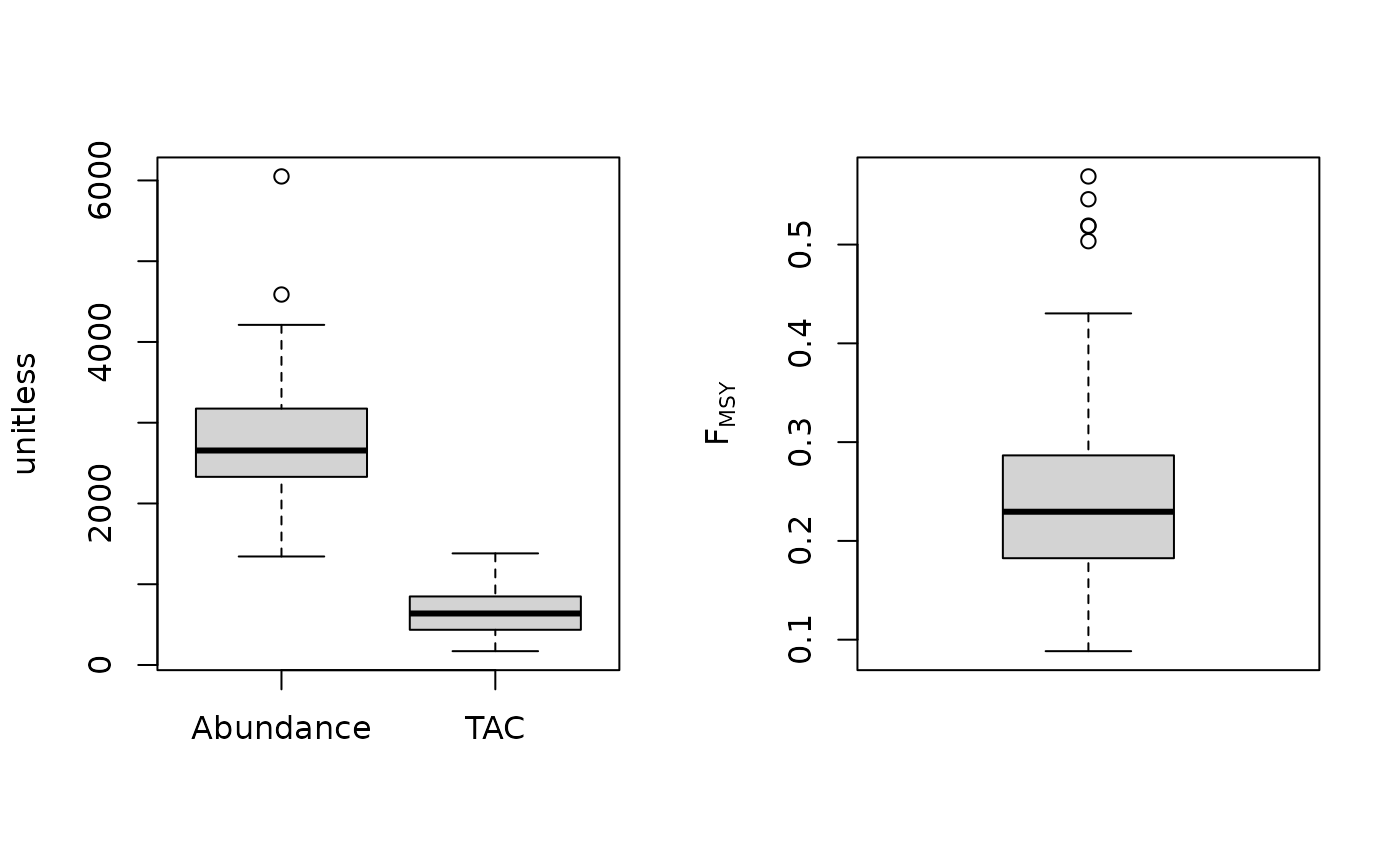FMSY is calculated as r/2 where r is calculated from a demographic approach (inc steepness). Coupled with an estimate of current abundance that gives you the OFL.

Fdem(x, Data, reps = 100, plot = FALSE)

Fdem_CC(x, Data, reps = 100, plot = FALSE, Fmin = 0.005)

Fdem_ML(x, Data, reps = 100, plot = FALSE, Fmin = 0.005)

## Arguments

x

A position in the data object

Data

A data object

reps

The number of stochastic samples of the MP recommendation(s)

plot

Logical. Show the plot?

Fmin

The minimum fishing mortality rate derived from the catch-curve analysis

## Value

An object of class Rec-class with the TAC slot populated with a numeric vector of length reps

## Details

The TAC is calculated as: $$\textrm{TAC} = F_{\textrm{MSY}} A$$ where A is an estimate of current abundance, and $$F_{\textrm{MSY}}$$ is estimated as $$r/2$$, where $$r$$ is the intrinsic rate of population growth, estimated from the life-history parameters using the methods of McAllister et al. (2001).

## Functions

• Fdem: Current abundance is assumed to be known (i.e Data@Abun)

• Fdem_CC: Current abundance is estimated from catch curve analysis

• Fdem_ML: Current abundance is estimated from mean length

## Required Data

See Data-class for information on the Data object

Fdem: Abun, FMSY_M, L50, MaxAge, Mort, steep, vbK, vbLinf, vbt0, wla, wlb

Fdem_CC: CAA, Cat, FMSY_M, L50, MaxAge, Mort, steep, vbK, vbLinf, vbt0, wla, wlb

Fdem_ML: CAL, Cat, FMSY_M, L50, Lbar, Lc, MaxAge, Mort, steep, vbK, vbLinf, vbt0, wla, wlb

## Rendered Equations

See Online Documentation for correctly rendered equations

T. Carruthers

## Examples

Fdem(1, MSEtool::SimulatedData, plot=TRUE)#> TAC (median)
#>     1352.567
Fdem_CC(1, MSEtool::SimulatedData, plot=TRUE)#> TAC (median)
#>     2002.109
Fdem_ML(1, MSEtool::SimulatedData, plot=TRUE)#> TAC (median)
#>     620.2194